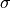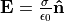# Image Charge Detection¶

Electrostatic induction is the effect of the charge on an object being redistributed upon placing other charge nearby it. Typically that object is a conductive electrode held to a constant potential, in which case the electron charges can freely move on the electrode surface, though only in a way that holds the electrode potential constant given changes to the nearby charge.

Image charge is the induced charge on an electrode. Changes to image charge over time, as due to changes to nearby charge, cause image current. (“Image current” may alternately be used in the sense of “method of images,” where the image charges are not real charges, but this is not precisely what is being discussed here.)

Image charge detection (or image current detection) is a method of detecting the presence of charge (or current) in space by measuring the charge (or current) induced on a nearby electrode. This is commonly used in FT-ICR (Penning Trap), where cyclotron motion of the trapped particles induces an image current on detection plates. This image current, as a function of time, can be passed through a Fourier transform to determine particle cyclotron frequencies, which in turn are related to m/z, thereby allowing the instrument to operate as a mass analyzer.

## SIMION-specific¶

Electrostatic induction occurs normally in SIMION as an indirect consequence of the Laplace Equation used in Refine. We typically might not think much of it though because unlike Coulomb’s Law, the Laplace Equation, and to lesser extent even the Poisson Equation, is expressed in terms of (constant) potentials rather than charges. However, we can readilly relate the electrode surface charge densityto the electric field E just above a section of the electrode surface via via equation. 

In general, observing image current, at least for high currents exhibiting Space Charge effects, may require invoking the Poisson Solver in SIMION throughout the trajectory integration (slow). However, for lower currents, there are efficient techniques (e.g. reciprocity) to measure image current in SIMION due to charged particle motion, as shown in SIMION Example: electrostatic_induction [8.1], with ICR as the typical application where this is used. Ideally, this should be measured at even time intervals (e.g. as can be forced with time markers) to permit an FFT.